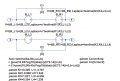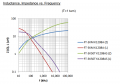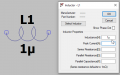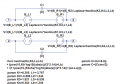# Frequency variable inductance in LTSpice

#### FuseFuse

Joined Feb 8, 2017
31
Hello,

I'm studying a SPICE model for common-mode chokes where windings are represented with frequency dependent voltage sources, as in the picture belowK and L parameters are fitted by measurement, while parallel capacitance is a fixed value.

I think it's a good way to simulated how magnetic permeability of a core varies with frequency, but I have some issue in setting the proper schematic to extract impedance curve.
What should I do? I'm used to define an external 1 V source (with 50 ohm series resistance) and a resistive 50 ohm load. Is it correct? I attached the corresponding LTspice file.

#### Attachments

• 2.5 KB Views: 2

#### ericgibbs

Joined Jan 29, 2010
17,180
hi FF,
E

#### FuseFuse

Joined Feb 8, 2017
31
hi FF,
E
This is a basical explaination on nominal behaviour of a common-mode choke. There's no modelling for frequency dependent permeability, that is you can only represent inductor with a constant slope impedance at low frequency and you can easily get it with an inductor, that's it.

What I'm looking for is to introduce a dependence of inductance on frequency. By a frequency-dependent source you define a Laplace transform, so you introduce a "frequency-like" variable (s).
In any case, the model I posted must have some issues since I can't extract expected impedance. Any hints?

#### Papabravo

Joined Feb 24, 2006
19,874
I know that permeability can be a function of magnetic induction (flux density) which is a function of current. What materials exhibit frequency dependent permeability?

#### FuseFuse

Joined Feb 8, 2017
31
I know that permeability can be a function of magnetic induction (flux density) which is a function of current. What materials exhibit frequency dependent permeability?
Permeability is frequency dependent, since it has an almost fixed DC value up to cut-off frequency, where permeability drops and capacitive behavior becomes predominant.
In particular, nanocrystalline cores show a frequency dependent inductance even at low frequencies. Let's have a look at this material for instanceBlue and green curves have a variable slope in the inductive region, so frequency-dependent inductances, that come from frequency-dependent permeabilities.
It's a bit tricky to reproduce this behavior in SPICE, since you can define one single inductive value for all the frequency range.The approach I showed before is interesting, as it introduces this L(f) function by using Laplace transforms.But the way this has to be properly used is unknown to me: I attached the .asc file with this schematic shown above. What components have to be connected to 1-4 label nets in order to get the blue or the green curve?

#### Attachments

• 2.5 KB Views: 0

#### Papabravo

Joined Feb 24, 2006
19,874
....
What should I do? I'm used to define an external 1 V source (with 50 ohm series resistance) and a resistive 50 ohm load. Is it correct? I attached the corresponding LTspice file.